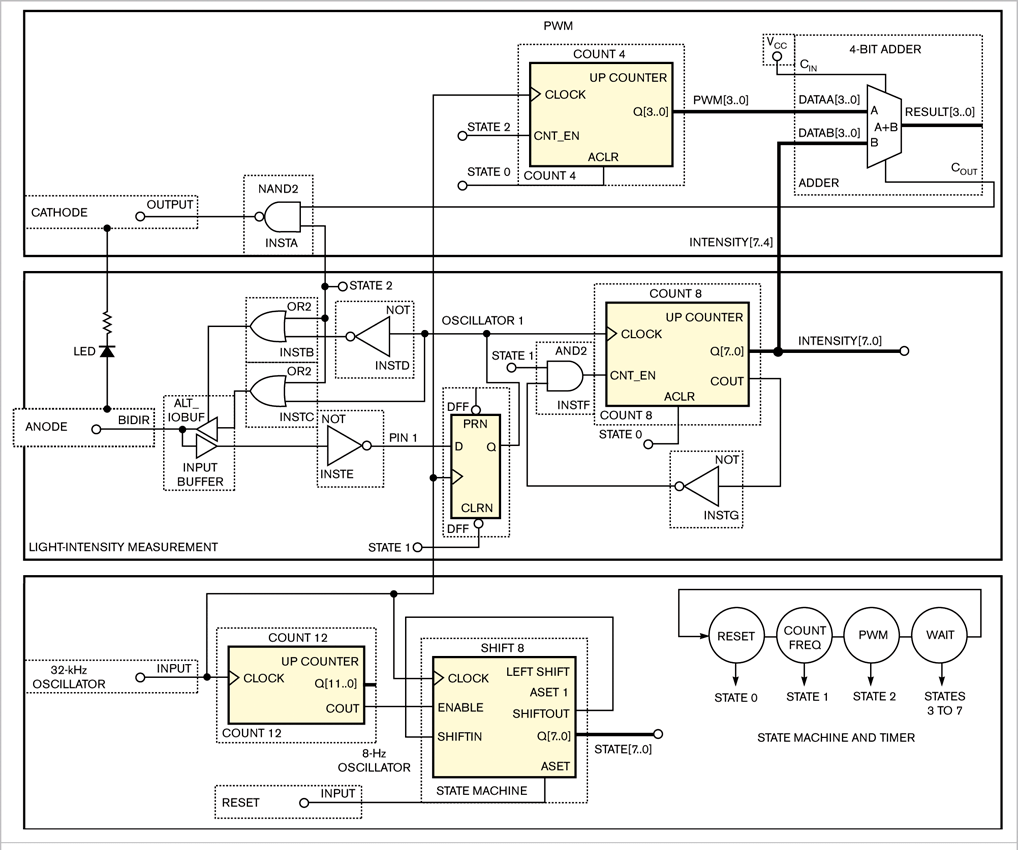# Use an LED to sense and emit light

## Altera EMP240

LEDs in portable devices often show power status, battery status, or Bluetooth-connection activity. LEDs can be major factors in determining battery life because their intensity is directly proportional to power drain. Using a simple circuit, the MAX IIZ CPLD from Altera can measure the analog-light level of its environment and then drive an LED at a proportional analog intensity level. A single LED can both sense and emit light with the same LED and bias resistor. The circuit in Figure 1 requires only 45 logic elements, and the programmability of the CPLD makes it easy to quickly adjust the parameters of the circuit to the characteristics of any LED.Click, for enlarge

Figure 1 This simple MAX IIZ circuit uses an LED as an emitter and a sensor.

You can reduce the power consumption of a flashing LED by increasing the flash period, decreasing the flash pulse width, or decreasing intensity. Controlling the LED intensity based on ambient light reduces LED energy usage by more than 47% without affecting appearance. Figure 1 shows a circuit that uses an Altera EMP240ZM100C7N CPLD, LED, resistor, and clock source to blink an LED with an intensity proportional to ambient light. The circuit comprises a PWM (pulse-width modulator) for driving the LED, a light-intensity-measurement block, and a controlling state machine and timer.

The state machine includes one hot state comprising an 8-bit shift register initialized to the 00000001 binary. The carryout of Count 12, a 12-bit counter, generates an 8-Hz enable signal for state machine Shift 8. Thus, each of the eight states of the state machine is active for 125 msec. In State 0, the reset state, PWM block and light-measurement block Count 8 are reset. State 1 is the light-intensity-measurement state, which enables a frequency counter, Count 8. Enabled for 125 msec, Count 8 counts the cycles exiting the light sensor. The circuit senses the light by biasing the LED and current-limiting resistor such that the cathode lead of the LED is at logic one. The anode connects to a relaxation oscillator that starts with anode at logic zero. The LED pulls up the anode in proportion to the amount of light hitting the LED. The reverse-biased LED acts as a solar cell with output current proportional to light. Once the slow-rising anode signal reaches the threshold of the input buffer, the Pin 1 signal becomes a zero, and the D flip-flop, DFF, toggles to zero and drives the anode signal to zero, making Pin 1 a logic one and tristating the input buffer on the next clock cycle, allowing the anode signal to rise again.

The frequency of Oscillator 1 is proportional to light intensity, with typical frequency for bright light of approximately 2000 Hz. The Oscillator 1 signal drives the clock of Count 8. Count 8 resets in State 0 and then is enabled in State 1 for 125 msec. In bright light, Count 8 might count to 250 at the end of the measurement, and, in low light, it might count to only 16. The counter’s COUT signal feeds back to the enable so that the count will saturate at a count of 255 and prevent high-intensity light from wrapping the counter back to zero and taking a false measurement.

State 2 is the LED’s blinking state. This state blinks the LED for 125 msec at an intensity that a PWM controls. In State 2, the cathode and anode pins are bias to the emitter mode. The emitter mode forces the anode signal to VCC. The cathode node connects to the PWM output. A logic zero on the cathode node lights the LED, and a logic one turns it off. The cathode signal is the inverted form of the PWM output.
In this example, the PWM is a 4-bit-resolution PWM, but you can use more or fewer bits. The PWM comprises binary counter Count 4 and a binary, 4-bit adder. The Count 4 counter is enabled in State 2, and the cycling output connects to the A input of the 4-bit adder. The B input of the adder connects to the four MSBs (most significant bits) of the light-sensor-frequency counter. The carryout of the adder is the PWM output. The carry-in of the adder is a constant logic one.

The following examples show how the PWM works:

• A logic zero from the intensity measurement results in a logic zero at carryout when Count 4 is zero through 14 and a logic one when Count 4 is 15. This 6.25% duty cycle is a very low-intensity level.
• A value of seven from the intensity measurement results in a logic zero at carryout when Count 4 is zero to seven and a logic one when Count 4 is eight to 15. This 50% duty cycle is a medium-intensity level.
• A 15 from the intensity measurement results in no logic zero at carryout for any Count 4 value and a logic one when Count 4 is zero through 15. This 100% duty cycle is a full-intensity level.

The only function of states 3 to 7 is to wait for the next LED-flash cycle. You can add or remove states to change the flash rate.

EDN